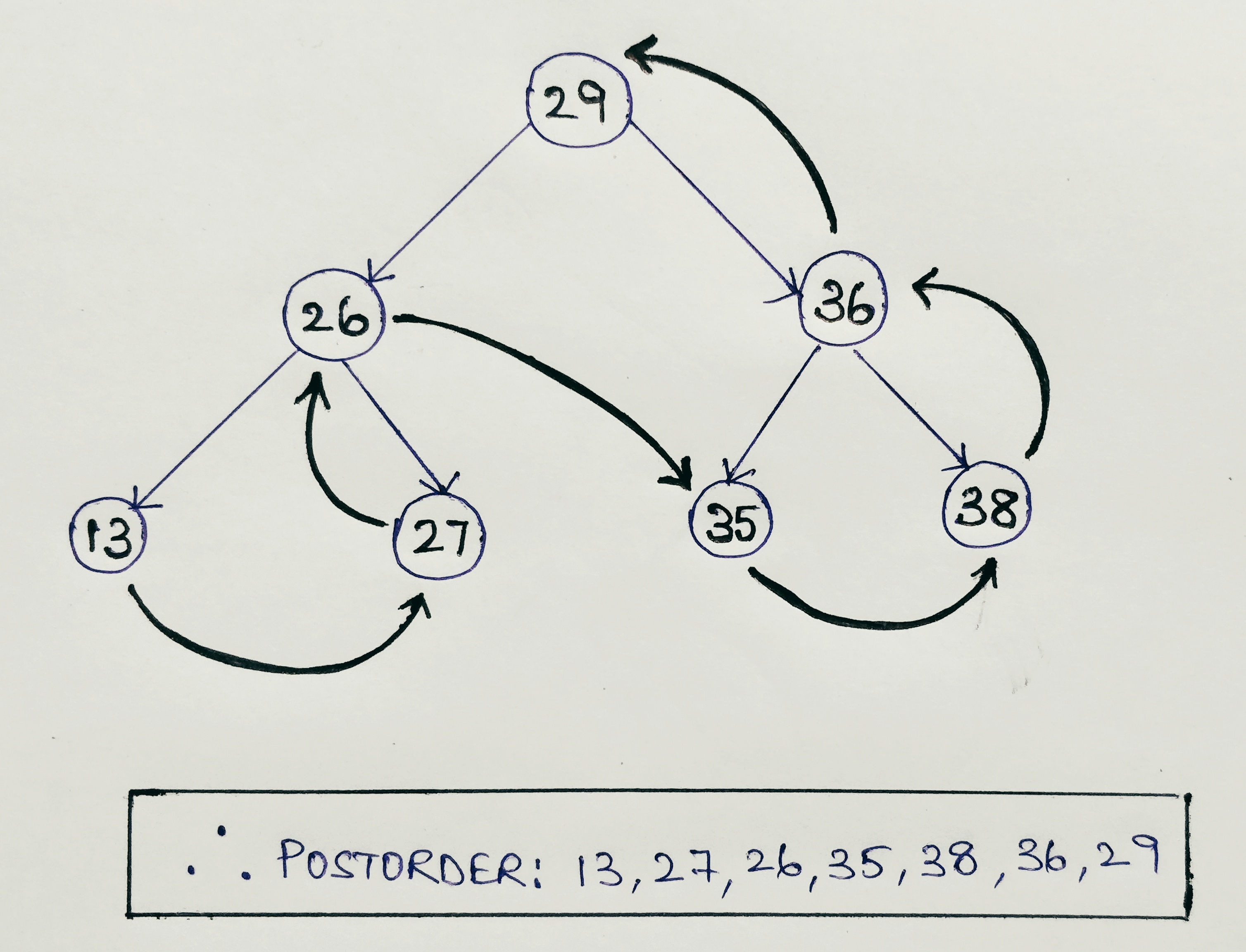Postorder tree Traversal using Recursion in Java

A hierarchical data structure like a tree can be traversed in many ways. In this tutorial, we will learn one of the three ways of DFS ( depth-first search ) that is Postorder Tree Traversal. It is also known as LRN ( Left -> Right -> Root ) algorithm. In this method, we visit the nodes of the left subtree, followed by the right subtree and then we visit the parent node in the end. Like all the other tree traversal algorithms the easiest way to implement postorder tree traversal is by using Recursion.

In depth-first search:

• If it is a graph, we select an arbitrary node as root and traverse across the nodes.
• If it is a tree, we start at the root and explore as far as possible along each branch before backtracking.

To traverse a tree in Postorder fashion there are three steps:
1. Visit all the nodes of the left subtree
2. Visit all the nodes of the right subtree
3. Visit the parent node

• We use postorder traversal to get the postfix expression of an expression tree.
• Unlike Inorder traversal which prints all nodes of binary tree in sorted order, Postorder doesn’t provide sorting but it is frequently used while deleting nodes from a binary tree as it deletes the child nodes first and then the parent nodes.

Note: If we traverse the right child before the left child then such a traversal is called Reverse Postorder traversal.The only difference between the Postorder tree traversal and Preorder tree Traversal is that, instead of printing the value of node first, we just call the recursive method on the left subtree, followed by the right subtree, as shown in the example.

To implement this in code we create a data structure to store a Binary Tree Node and then we write a function to create a new binary tree node having given value.

class Node
{
int data;
Node left, right;
public Node(int value)
{
data = value;
left = right = null;
}
};

Code for Postorder Traversal with recursion in Java

class Node
{
int data;
Node left, right;
public Node(int value)
{
data = value;
left = right = null;
}
};

class PostorderTraversal
{
public static void postorder(Node root)
{
if (root == null) {
return;
}

postorder(root.left);
postorder(root.right);
System.out.print(root.data + " ");
}

public static void main(String[] args)
{
Node root = new Node(5);
root.left = new Node(10);
root.right = new Node(16);
root.left.left = new Node(6);
root.left.right = new Node(2);
root.right.left = new Node(3);
root.right.right = new Node(1);

System.out.print("The postorder traversal for the tree is: ");
postorder(root);
}
}

Output:

The postorder traversal for the tree is: 6 2 10 3 1 16 5

Explanation

• Firstly we construct the tree and use the recursive function to perform Postorder traversal of the tree.

The Constructed tree is:

5
/    \
/        \
10           16
/ \           / \
/      \      /     \
6       2    3       1

• If the current node is not empty :
1. Traverse the left subtree recursively and display the node.
2. Traverse the right subtree recursively and display the node.
3. Display the parent node.

Hope you’ve understood the code 🙂
Any questions please feel free to drop in your comments.

You can also read: